• Call Now

1800-102-2727•

# Mohr’s Salt Titration with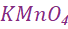- Introduction, Mohr’s Salt, Theory of Estimation of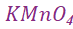, Materials Required, Procedure, Practice Problems, FAQs

Chemistry is colour. Chemical compounds and their aqueous solutions exhibit a variety of fascinating colours. One such substance is potassium permanganate. But we do not stop admiring these colours we are interested in their qualitative and quantitative properties.

Titration is one such procedure for estimating the strength or amount of a substance. Substances react proportionally to their equivalent weight.

Generally, external indicators are used in the titration process to predict the completion of colourless solutions reactions. Potassium permanganate solution is quite pink in colour and hence its presence or absence can be easily identified by the presence or absence of the pink colour. That means, no external indicator will be needed in titrations involving potassium permanganate.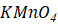act as a self-indicator. At the equivalence point of the titration, it can be observed that the deep purple colour of permanganate changes to a faint pink colour or colourless.

Here we shall see the estimation ofin the solution by titrating it against a ferrous ammonium sulphate reference solution. Yes, the titration we going to study is titration ofwith Mohr’s salt. We will study the process, methods and application in detail.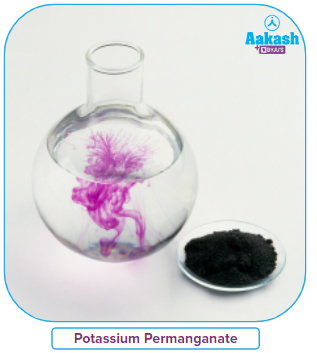TABLE OF CONTENT

• What is Mohr’s Salt?
• Theory of Estimation of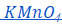• Materials Required
• Procedure
• Calculation
• Practice Problems

What is Mohr’s salt?

It should be noted that German scientist Karl Friedrich Mohr inspired the name of Mohr's salt. Mohr's salt is a double salt containing ferrous sulphate and ammonium sulphate with six moles of water of crystallisation. Like the majority of other ferrous sulphate salts, it dissolves in water to create an aqueous complex having the chemical formula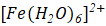. It should be noted that the molecular geometry of this aqua complex is octahedral. Mohrite is the name for Mohr's salt in its mineral form.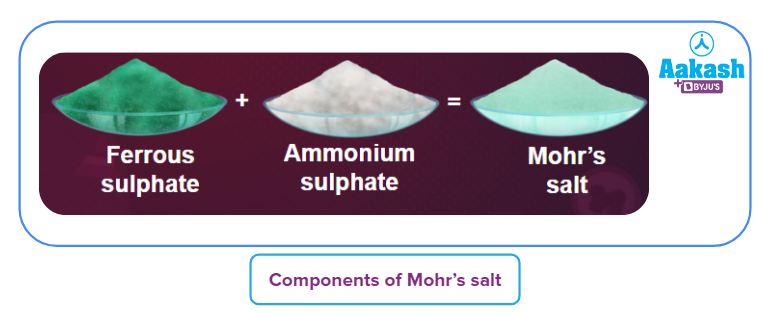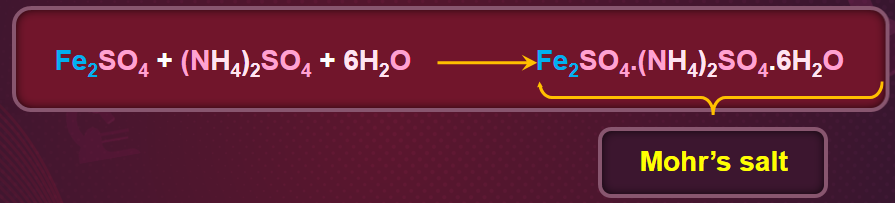Theory of Estimation of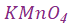Both potassium permanganate and ferrous ammonium salt is used for preparing standard solutions. Any one of these solutions of known concentration can be used to estimate the concentration (strength) of the other solution of unknown concentration.

Ferrous ions in the Mohr’s salt, can be oxidized to ferric ions and hence the Mohr’s salt acts as a reducing agent. Ammonium sulphate present along with ferrous ions does not undergo redox reactions and hence is a mute spectator.

Potassium permanganate is a well-known oxidizing agent, particularly in an acid medium.

Hence mixing potassium permanganate and Mohr’s salt undergoes reduction-oxidation reactions.

The following list describes the reaction that occurs:

1. Chemical equation:

Reduction half-reaction: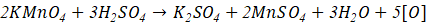Oxidation half-reaction: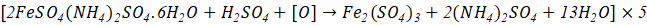Overall reaction: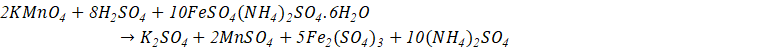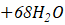1. Ionic equation:

Reduction half-reaction: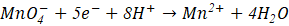Oxidation half-reaction: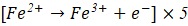Overall reaction: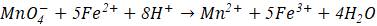Two moles of potassium permanganate reacts with ten moles of Mohr’s salt.

Note:

Mohr's salt contains iron with an oxidation value of +2. Iron undergoes oxidation throughout the reaction, changing from +2 to +3. Because the reaction rate is so high even at room temperature, heating the ferrous ammonium sulphate solution is not necessary for this titration.

Additionally, at high temperatures, air oxygen can convert ferrous ions to ferric ions, which could cause mistakes in the experiment.

The same titration can be used to estimate the unknown concentration of Mohr’s salt using standard potassium permanganate solution

Materials Required:

Below are the materials required to initiate the experiment.

• Mohr’s salt (ferrous ammonium sulfate)
• Potassium permanganate solution
• Dilute sulfuric acid
• Burette
• Chemical balance
• Pipette
• Burette stand
• Funnel
• Weighing bottle
• White tile

Procedure:

1. Making a 0.05 M ferrous ammonium sulphate standard solution:

(Molar mass of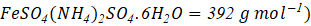.

• Weigh 4.9000 g of ferrous ammonium sulphate in a weighing bottle and transfer the contents via a funnel into a 250 mL standard flask.
• Pour distilled water into the flask to transfer the solid that is stuck to the funnel, and then add diluted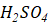drop by drop to create a clear solution. Make up the solution to the mark in the standard flask.
• Shake the flask until the material dissolves.

2. Titration of potassium permanganate solution with ferrous ammonium sulphate.

• Rinse the burette with potassium permanganate solution and then fill it up. By releasing some solution through the burette tip, remove any air bubbles that may be present. The zero mark of the burette should always coincide with the upper meniscus of the potassium permanganate.
• Place half a test tube's worth of (1.0 M)in a conical flask.
• Pipette out exactly 10 mL of 0.05 M ferrous ammonium sulphate solution into the conical flask.
• Add potassium permanganate solution to the aforementioned conical flask solution slowly with continuous shaking, until the solution's colour turns permanently pink. The endpoint is the appearance of a permanent pink colour.
• Repeat the titration until you get three readings that are in agreement.
• Write down the readings according to observation, then convert them into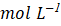to determine the strength of the potassium permanganate solution.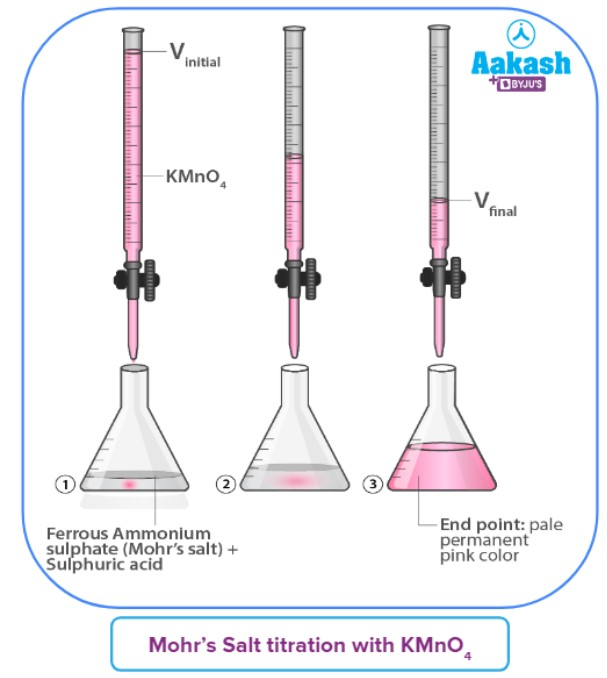Calculations:

The following equation can be used to calculate the molarity and strength of an unknown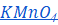solutions: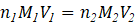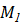= Molarity of ferrous ammonium sulphate.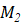= Molarity of potassium permanganate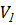= Volume of ferrous ammonium sulphate.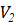= Volume of potassium permanganate.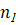= 1, (n is the n-factor equal to the number of electrons lost per formula unit during redox reactions. Here for the ferrous ammonium sulphate, n =1, in the half cell reaction)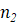= 5, (the number of electrons gained per formula unit of potassium permanganate in a half cell reaction)

The formula below can be used to calculate strength:

Molarity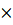Molar mass = Strength = amount present

Using above calculation method one can easily calculate the strength of potassium permanganate solution.

Precautions:

1. Consistently keep the flask, pipette, and burette clean.

2. Before doing the experiment, thoroughly clean all of the equipment.

3. To do the experiment, use diluted acid.

4. Don't keep filling the burette with potassium permanganate solution.

5. After adding potassium permanganate solution, be sure to shake the conical flask.

6. Stop adding potassium permanganate solution until the solution turns permanently pink and does not vanish when shaken.

7. To ensure the accuracy of the experiment, always take at least three sets of readings.

Practice Problems:

1. The number of phases in Mohr salt is__________

A. 2

B. 3

C. 0

D. 1

Solution: Mohr salt is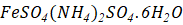. Since it is a single component (though it has many species), it has only one phase (a component with n species has only one compound).

2. Oxidation state of iron in Mohr’s salt is calculated as_______.

A. +2

B. +3

C. 0

D. +4

Solution: The given compound i.e Mohr’s salt is.

Let us suppose the oxidation state of Fe be x.

The oxidation number of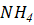is +1

The oxidation number of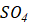is −2

The oxidation number of H is +1

The oxidation number of O is −2

The oxidation number of Fe inis calculated as,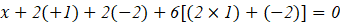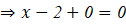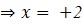Hence, the oxidation state of iron comes out to be +2 in3. Potassium permanganate is used as a self-indicator because of __________

A. It behaves as a strong oxidising agent

B. It breaks down into ions easily

C. It gets disproportionated in acidic medium

D. It undergoes a chemical change resulting in colour difference.

Solution: Potassium permanganate has a rich violet colour that is extremely visible to the human eye, and when it is titrated, there is a noticeable loss of colour.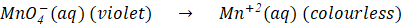4. A 0.05 N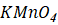solution required 55 mL of 0.067 N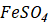for complete reaction in an acidic medium. Calculate the volume ofsolution required.

A. 7.4 mL

B. 740 mL

C. 74 mL

D. None of these

Solution:

We know that in an acidic medium,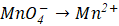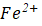is oxidised to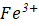Overall reaction: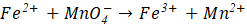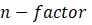of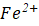=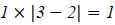of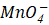=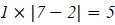We know that Normality = Molarityn-factor

Number of equivalent = NormalityVolume (L)

Number of equivalent = Molen-factor

According to the law of equivalence, Equivalent of= Equivalent of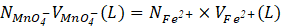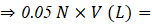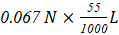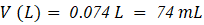So, option C is the correct answer.

1. What uses do you have for Mohr's salt?
Mohr's salt is a well-known source of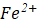ions in the realm of analytical chemistry. Because it has a reasonably long shelf life and is resistant to oxidation brought on by contact to the atmosphere, Mohr's salt is a good source of ferrous ions. Fricke's dosemeter, which aids in the measurement of large doses of gamma radiation, also uses Mohr's salt.

2. Why does titration use Mohr's salt?
Mohr's salt is known as ferrous ammonium sulphate chemically. Mohr salt serves as a reducing agent and potassium permanganate as an oxidising agent in this titration, therefore used as redox titration.
It is a stable salt.

3. Why is diluted sulfuric acid added to Mohr's salt aqueous solutions?
To stop the hydrolysis of ferrous sulphate, diluted sulphuric acid is often added to the aqueous solutions of Mohr's salt.

4. In Mohr salt, what kind of solution is employed in titration?
A tiny amount of sulfuric acid and an equal mixture of hydrated ferrous sulphate and ammonium sulphate are combined with water to create Mohr's salt. Now, crystallisation is applied to the resulting solution mixture. After that, we obtain tiny Mohr's salt crystals that are light green in colour.

Related Topics:Talk to our expert
Resend OTP Timer =
By submitting up, I agree to receive all the Whatsapp communication on my registered number and Aakash terms and conditions and privacy policy